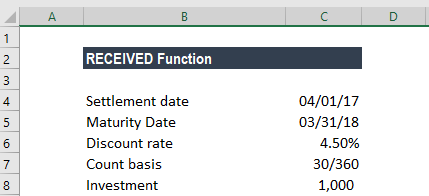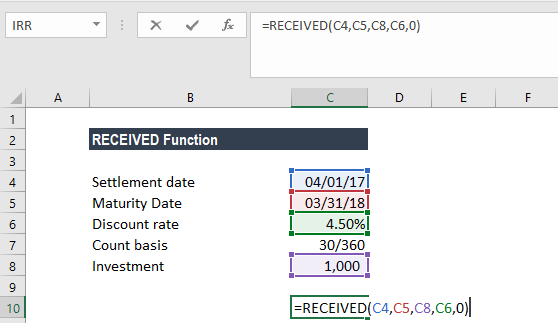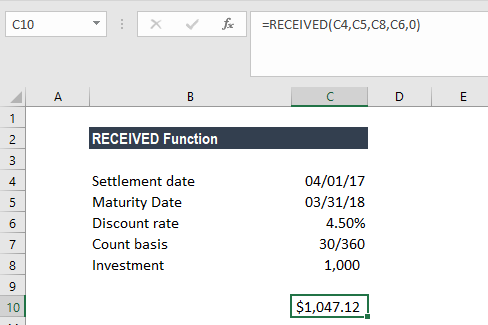Calculate the amount received at maturity for a fully invested security

## What is the Maturity RECEIVED Function?

The RECEIVED function is categorized under Excel Financial functions. It will calculate the amount received at maturity for a fully invested security.

As a financial analyst, the RECEIVED function helps calculate the amount that we will get from an investment if the duration of the investment and interest rate are given.

The RECEIVED function uses the following arguments:

1. Settlement (required argument) – This is the security’s settlement date. It is the date after the issue date when the security is traded to the buyer.
2. Maturity (required argument) – The security’s maturity date or when it expires.
3. Investment (required argument) – This is the amount invested in the security.
4. Discount (required argument) – This is the security’s discount rate.
5. Basis (optional argument) – The type of day count basis to be used.

BasisDay Count basis
0 or omittedUS(NASD) 30/360
1Actual/actual
2Actual/360
3Actual/365
4European 30/360

#### Notes

• Excel stores dates as sequential serial numbers so they can be used in calculations. By default, January 1, 1900 is serial number 1, and January 1, 2018 is serial number 43101 because it is 43,101 days after January 1, 1900.
• Settlement, maturity, and basis are truncated to integers.

### How to use the maturity RECEIVED Function in Excel?

To understand the uses of the function, let’s look at an example:

#### Example

Suppose we are given the following data:Calculate the amount received at maturity on an investment of \$1,000, which was used to purchase a security on April 1, 2017. The security matured on March 31, 2018, with a discount rate of 4.5%. The US (NASD) 30/360 day count basis is used:

The formula used is:We get the result below:In the above example:

• As recommended by Excel, the settlement and maturity dates are provided to the function as references to dates stored in cells B2 and B3.
• The discount argument is supplied to the function as a percentage value, 4.5%. It is also possible to provide the argument as the decimal value 0.045.
• The [basis] argument is omitted and so takes on the default value of 0 and, therefore, uses the US(NASD) 30/360 basis.

1. #VALUE! error – Occurs if:
• Any of the given arguments are non-numeric.
• The settlement or maturity arguments provided are not valid Excel dates.
2. #NUM! error – Occurs if:
• The investment or discount argument provided is less than or equal to zero.
• The basis argument given is not equal to 0,1,2,3, or 4.
• The given maturity date is less than or equal to the settlement date.

Thanks for reading CFI’s guide to important Excel functions! By taking the time to learn and master these functions, you’ll significantly speed up your financial modeling and analysis. To learn more, check out these additional CFI resources:

• Excel Functions for Finance